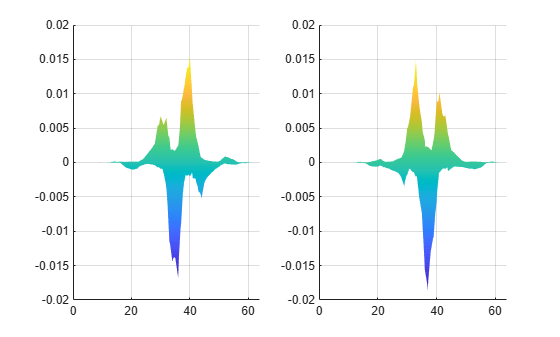# idualtree3

3-D dual-tree complex wavelet reconstruction

## Syntax

``xrec = idualtree3(a,d)``
``xrec = idualtree3(a,d,Name,Value)``

## Description

example

````xrec = idualtree3(a,d)` returns the inverse 3-D dual-tree complex wavelet transform of the final-level approximation coefficients, `a`, and cell array of wavelet coefficients, `d`.```
````xrec = idualtree3(a,d,Name,Value)` specifies options using name-value pair arguments.```

## Examples

collapse all

Generate all-zero sets of scaling and wavelet coefficients by computing the 3-D dual-tree complex wavelet transform of an array of zeros.

```zr = zeros(64,64,64); [a,d] = dualtree3(zr,4);```

Find the real (4,5) wavelet coefficient of the 19th subband of the third level by assigning 1 to the corresponding array element and inverting the transform.

```d{3}(4,5,19) = 1; xr = idualtree3(a,d);```

Find the corresponding imaginary coefficient assigning the imaginary unit to the array element and then inverting the transform.

```[a,d] = dualtree3(zr,4); d{3}(4,5,19) = 1j; xi = idualtree3(a,d);```

Display the 18th page of the real and imaginary reconstructions.

```subplot(1,2,1) surf(xr(:,:,18)) view(0,0) zlim([-0.02 0.02]) shading interp subplot(1,2,2) surf(xi(:,:,18)) view(0,0) zlim([-0.02 0.02]) shading interp```## Input Arguments

collapse all

Final-level scaling coefficients, specified as a real-valued matrix. `a` is an output of `dualtree3`.

Data Types: `single` | `double`

Wavelet coefficients, specified as a cell array. `d` is an output of `dualtree3`.

Data Types: `single` | `double`
Complex Number Support: Yes

### Name-Value Pair Arguments

Specify optional comma-separated pairs of `Name,Value` arguments. `Name` is the argument name and `Value` is the corresponding value. `Name` must appear inside quotes. You can specify several name and value pair arguments in any order as `Name1,Value1,...,NameN,ValueN`.

Example: `'LevelOneFilter','legall','FilterLength',6` inverts a transform using LeGall synthesis filters with scaling length 3 and wavelet length 5 at level 1, and length-6 Q-shift filters at levels 2 and greater.

Hilbert Q-shift filter-pair length, specified as the comma-separated pair consisting of `'FilterLength'` and one of 6, 10, 14, 16, or 18. The synthesis filters used by `idualtree3` must match the analysis filters used by `dualtree3`.

Data Types: `double` | `single`

First-level biorthogonal analysis filter, specified as the comma-separated pair consisting of `'LevelOneFilter'` and a character vector or string. By default, `idualtree3` uses the near-symmetric biorthogonal wavelet filter with lengths 7 (scaling synthesis filter) and 5 (wavelet synthesis filter) in the reconstruction.

Data Types: `char` | `string`

Size of the original data, specified as the comma-separated pair consisting of `'OriginalDataSize'` and a three-element vector of even integers. This vector must match the size of the original input to the 3-D dual-tree wavelet transform. When the first-level wavelet coefficients are not available, the reconstructed data size can differ from the original input data size. If you call `dualtree3` with the `'excludeL1'` option, then `'OriginalDataSize'` adjusts the size of `xrec` to match the size of the original input data. If you do not use the `'excludeL1'` option, then this argument is ignored.

Data Types: `double` | `single`

## Output Arguments

collapse all

Inverse 3-D dual-tree complex wavelet transform, returned as a 3-D array.

 Chen, H., and N. G. Kingsbury. “Efficient Registration of Nonrigid 3-D Bodies.” IEEE® Transactions on Image Processing. Vol 21, January 2012, pp. 262–272.

 Kingsbury, N. G. “Complex Wavelets for Shift Invariant Analysis and Filtering of Signals.” Journal of Applied and Computational Harmonic Analysis. Vol. 10, May 2001, pp. 234–253.

## Support